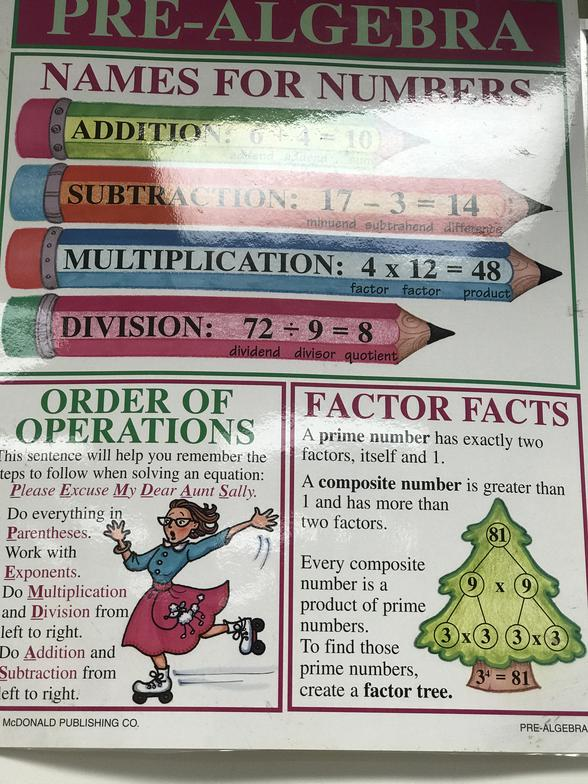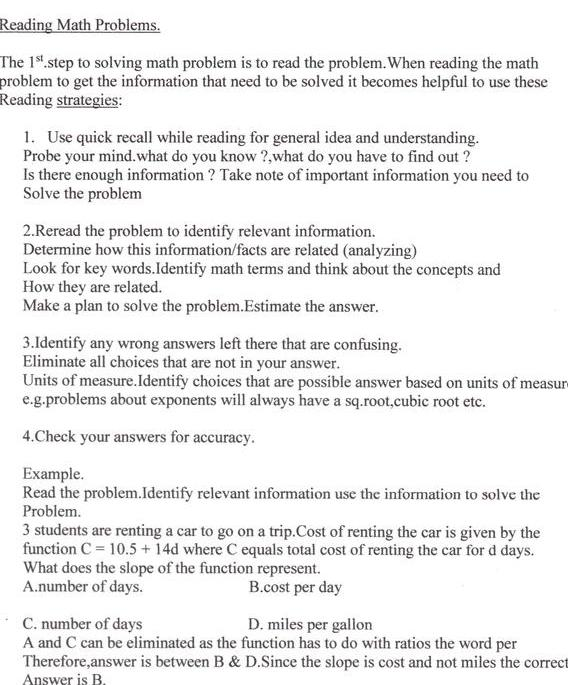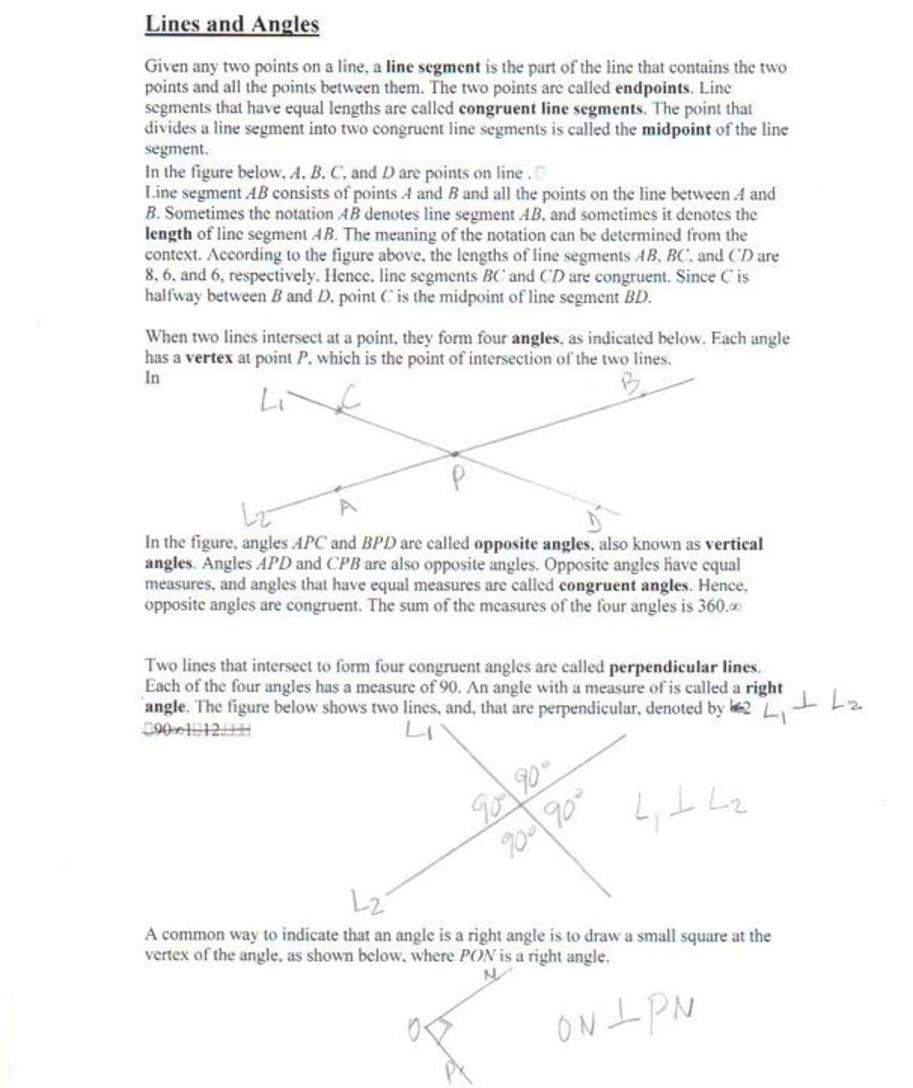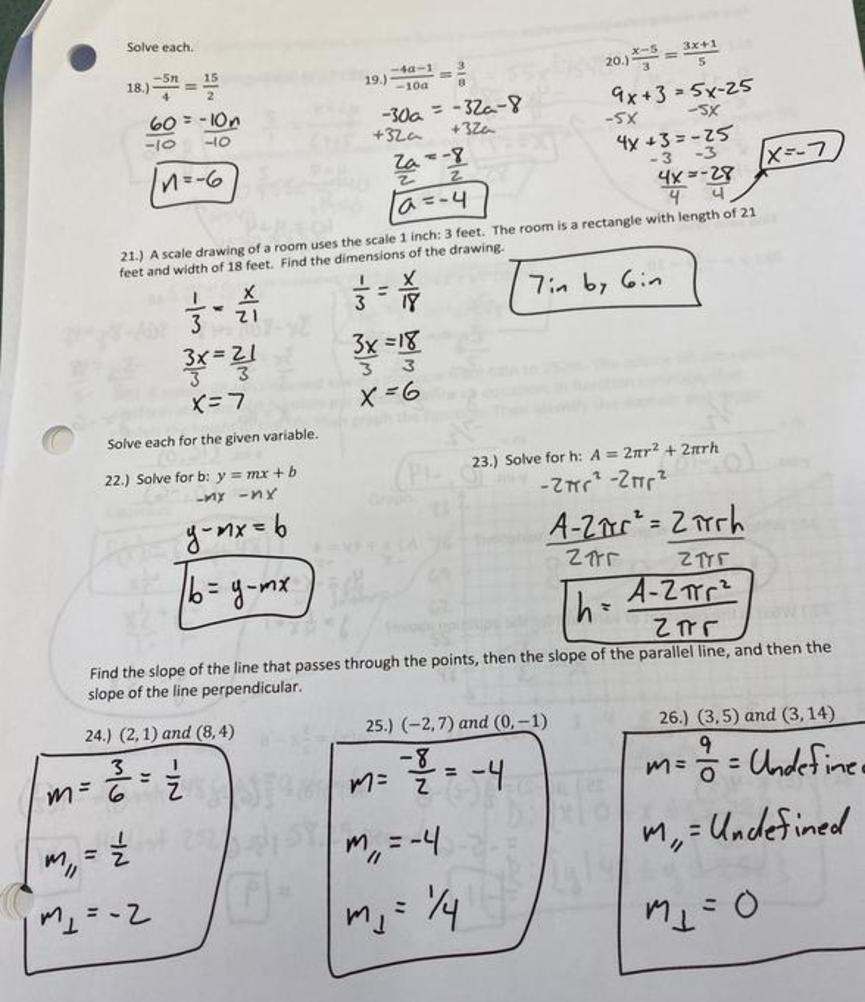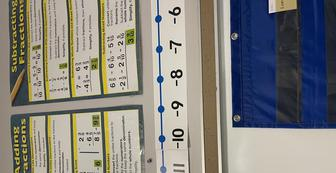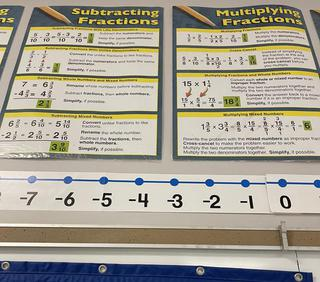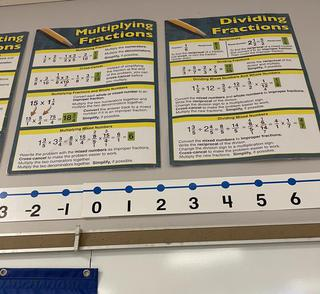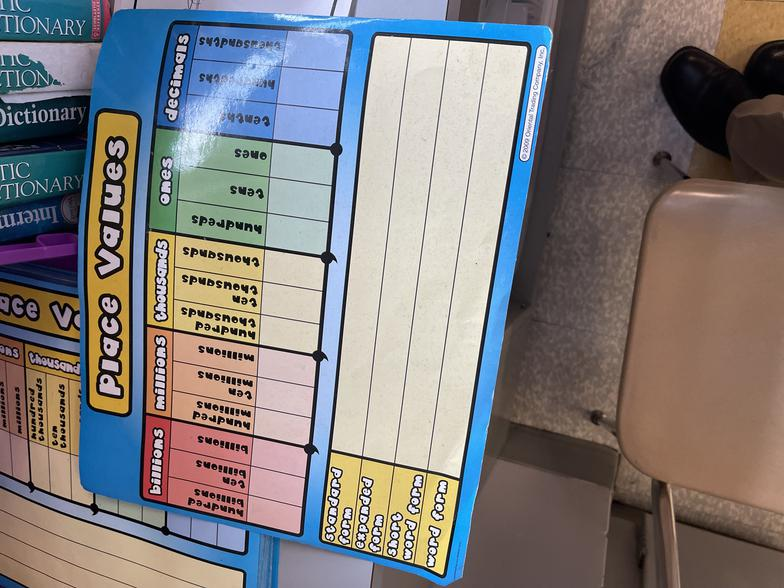hopeforchildren foundation inc. is happy to coordinate all of our related services with our Partners.

​                            btthemathpage@nyc.rr.comhemathpage@nyc.rr.com
SOME RULES

OF
ALGEBRA

The rule of symmetry

The commutative rules

Two rules for equations

ALGEBRA, we can say, is a body of formal rules. They are rules that show how something written one form may be rewritten in another form. For what is a calculation if not transforming one set of symbols into another? In arithmetic we may replace the symbols '2 + 2' with this symbol '4.' In algebra, we may replace 'a + (−b)' with 'a − '

Here are some of the basic rules of algebra:1· a=a.(1 times any number does not change it. Therefore 1 is called the identity of multiplication.)(−1)a=−a.−(−a)=a.(Lesson 2)a + (−b)=a − b.(Lesson 3)a − (−b)=a + b.(Lesson 3)Associated with these -- and with any rule -- is the rule of symmetry:

If a = b, then b = a.

For one thing, this means that a rule of algebra goes both ways.

Since we may write
p + (−q)=p − q

-- that is, in a calculation we may replace p + (−q) with p − q -- then, symmetrically:
p − q=p + (−q).

We may replace p − q with p + (−q).

The rule of symmetry also means that in any equation, we may exchange the sides.
If15=2x + 7,

then we are allowed to write2x + 7=15.

And so the rules of algebra tell us what we are allowed to write. They tell us what is legal.

​A statement of equality between two algebraic expressions that is true for only certain values of the variables involved is called an equation. The values are called the solutions of the equation.

​The following are examples of some basic types of equations.

3x + 5 = -2  A Linear equation in one variable, x

x -3y = 10  A Linear equation in two variables,x and y

2
20y + 6y - 17 = 0 A quadratic equation in one variable, y

Rules of Exponents:
a
​In the algebraic expression x  where x is raised to the power a, x is called the base and a is called the exponent.
For some equations involving bases and exponents, the following property is very useful:
y                         6
For Example: If  2 = 64 since 64 = 2 , we have

y    6
2 = 2     and can conclude that y = 6

Some basic rules of exponents:

- a     1
x   = ------             a      1
a    and  2    = ----
x                         -a
2
a    b        a + b
(x ) (x )  = (x        )
a
x         a - b       1
__  =  x        =   ___
b                        b - a
​x                        x

The commutative rules

The order of terms does not matter. We express this in algebra by writing

a + b = b + a

That is called the commutative rule of addition. It will apply to any number of terms.

a + b − c + d = b + d + a − c = −c + a + d + b

The order does not matter.

Example 1. Apply the commutative rule to p − q.

Solution. The commutative rule for addition is stated for the operation + . Here, though, we have the operation − . But we can write

p − q = p + (−q).
Therefore,
p − q = −q + p.

Here is the commutative rule of multiplication:

ab = ba

The order of factors does not matter.

abcd = dbac = cdba.

The rule applies to any number of factors.

What is more, we may associate factors in any way:

​Solving Linear Equations:

A linear equation is an equation involving one or more variables in which each term in the equation is either a constant term or a variable multiplied by a coefficient. None of the variables are multiplied together or raised to a power greater than 1.

For example,2x + 1 = 7x  and 10x - 9y - z = 3

Linear Equations in One Variable:
To solve a linear equation in one variable, simplify each side of the equation by combining like terms. Then use the rules for producing simpler equivalent equations.

11 x - 4 - 8 x = 2 (x - 4 ) - 2 x

3x - 4   =  2x  + 8 - 2 x    (like terms combined)

3x - 4  =  8                    (Simplified)

3x - 4  + 4  =  8 + 4               (4 added to both sides)

3x = 12

3x  =  12
___     ___                        (Both sides divided by 3)

3         3

Linear Equations in Two Variables:

There are two basic methods for solving systems of linear equations, by substitution or by elimination. In the substitution method, one equation is manipulated to express one variable in terms of the other. Then the expression is substituted in the other equation. For example, to solve the system of equations.

4 x + 3 y = 13
x  + 2y  =  2

we can express x in the second equation in terms of y, x = 2 - 2y then substitute 2 - 2y for x
in the first equation to find the value of y.

4(2 - 2y) + 3y = 13

8 - 8y + 3y =  13

- 8y + 3y =  5   ( 8 subtracted from both sides)

- 5y = 5    (like terms combined)

y = - 1  (both sides divided by - 5)

And - 1 can be substituted for y in either equation to find the value of x .Using second equation

x + 2 y  = 2

x + 2 (- 1) = 2

x - 2 = 2  now add 2 to both sides to get value of  x

​In the elimination method, the object is to make the coefficients of one variable the same in both equations so that one variable can be eliminated either by adding the equations together or by subtracting one from the other.
​Using the same example,multiply both sides of the equation 4 yields 4(x = 2y) or 4x + 8y = 8
now we have 2 equations with the same coefficient of x

4x + 3y = 13
4x + 8y =  8
subtract second equation from the first thus, x = 4 , y = - 1

 HomeAbout UsServicesDONATIONSProductsScience PageMath PageTechnologyJobsMath page 1Reading & WritingPHYSICSCHEMISTRYBIOLOGYSANDY HOOK ELEMENTARYVIDEOSMath Page 2

A quadratic equation in the variable x is an equation that can be written in the form
2
ax + bx + c = 0

where a, b, and c are real numbers and a =/= 0 When such an equation has solutions, they can be found using the
2
x == _- b ± _sqroot b_ -4ac
_____________________
2a

Where the notation ± is shorthand for indicating two solutions one that uses the plus sign and the other that uses the minus sign. ±
2
Example: In the quadratic equation 2x – x – 6 = 0

We have a = 2, b = - 1 and c = -6

X = -(-1) ± Sq.Root (-1) – 4 (2) (-6)
--------------------------------------------------
2 (2)

=         1 ± Sq Root 49
-----------------------------

4
=                1 ± 7
--------
4
Hence, the two solutions are : x = 1 + 7
---------- = 2
4

and   x  =  1 - 7          3
---------  = --------
4            2

Factorization is simplifying a quadratic equation
into 2 brackets.

2
Y  = X + 4X + 3  .........(1)

Y = (X + 1) ( X + 3) .......(2)

Solving a quadratic equation involves finding
2 values of X that make equation = 0

In the example:

2
X + 4X + 3 = 0

(X + 1) ( X + 3) = 0
Since X + 1 = 0; X = - 1 and X + 3 0, = -3
This is true as the 2 equations are a factor of 0
their products.Solved by finding the 2 roots.

An algebraic expression has one or more variables and can be written as a single term or as a sum of terms. Here are some examples of algebraic expressions.
3          2   2
2x ; y - 1/4 ; W  Z - 5 Z - Z + 6 ; 8/n - p

In the examples above, 2x is a single term,

y - 1/4 has two terms,
​
3          2   2
W  Z - 5 Z - Z  + 6 has four terms, and

8/n - p has one term.
​                              3         2    2
In the expression W  Z - 5 Z - Z + 6
2          2
5 Z and - Z  are called like terms because they have the same variables (Z), and the corresponding variables have the same exponents (2).
A term that has no variable is called a constant term.
what's the constant term in the above expression ?

A number that is multiplied by variables is called the coefficient of a term.                                    2
For example, in the expression 2 X + 7 X - 5
2
2 is the coefficient of the term 2 X  and 7 is the coefficient of the term 7 X and - 5 is a constant term.

The same rules that govern operations with numbers apply to operations with algebraic expressions.
One additional rule, which helps in simplifying algebraic expressions, is that like terms can be combined by simply adding their coefficients, as the following examples show.
3          2    2          3          2
2 X + 5X = 7X and W Z + 5 Z - Z + 6 = W Z + 4 Z + 6

3xy + 2x - xy -3x = 2xy - x

Factorization:
A number or variable that is a factor of each term in an algebraic
expression can be factored out as in the following examples.
2
4x + 12 = 4(x + 3) and 15y - 9y = 3y(5y - 3)

To multiply two algebraic expressions,each term of the first
expression is multiplied by each term of the second expression
and the results are added.As in the following examples.

(x + 2)(3x - 7) = x(3x) + x(- 7) + 2(3x) + 2(- 7)

2                                     2
= 3x - 7x + 6x - 14 which  = 3x  - x - 14

LESSON 2.

HOW TO FACTORIZE AND SOLVE QUADRATIC EQUATIONS.

​Consider factorizing only quadratic equation where the co-efficient of the

2                          2
X term , a = 1 and X + bX + C = 0

Question:Factorize and solve the quadratic equation below;

2
X + 5X + 4  = 0
Factorization: (X + X ) (X + X ) = 0
1           2

Step 1.Find the values of a,b,&c where a =1;b = 5; c = 4

step 2.The c term is the product of the roots  c = x   x
1   2

List the pair of factors that multiply to make the c term: c = 4 (1 x 4 ) (2 x 2 )

Step 3.b term is the sum of the roots x    x  = 5  therefore b = 5 (1 +4) = 5
1    2
Step 4.Insert the values in the pair of brackets x  = 1; x  =  4 (x+10 (x + 4) = 0
1         2
Solution:equate the 1st brackets  = 0 using substitution method to find x
1
x  = 1 = 0  therefore,x  = -1  Equate the 2nd bracket x  +  4 = 0 ;x  =  -4
1                            1        2                                    2               2
the solution of the equation x + 5x + 4 = 0 is x = -1; or x = -4
HOME SCHOOL ONLINE

Attend a home school online at the comfort of your home.